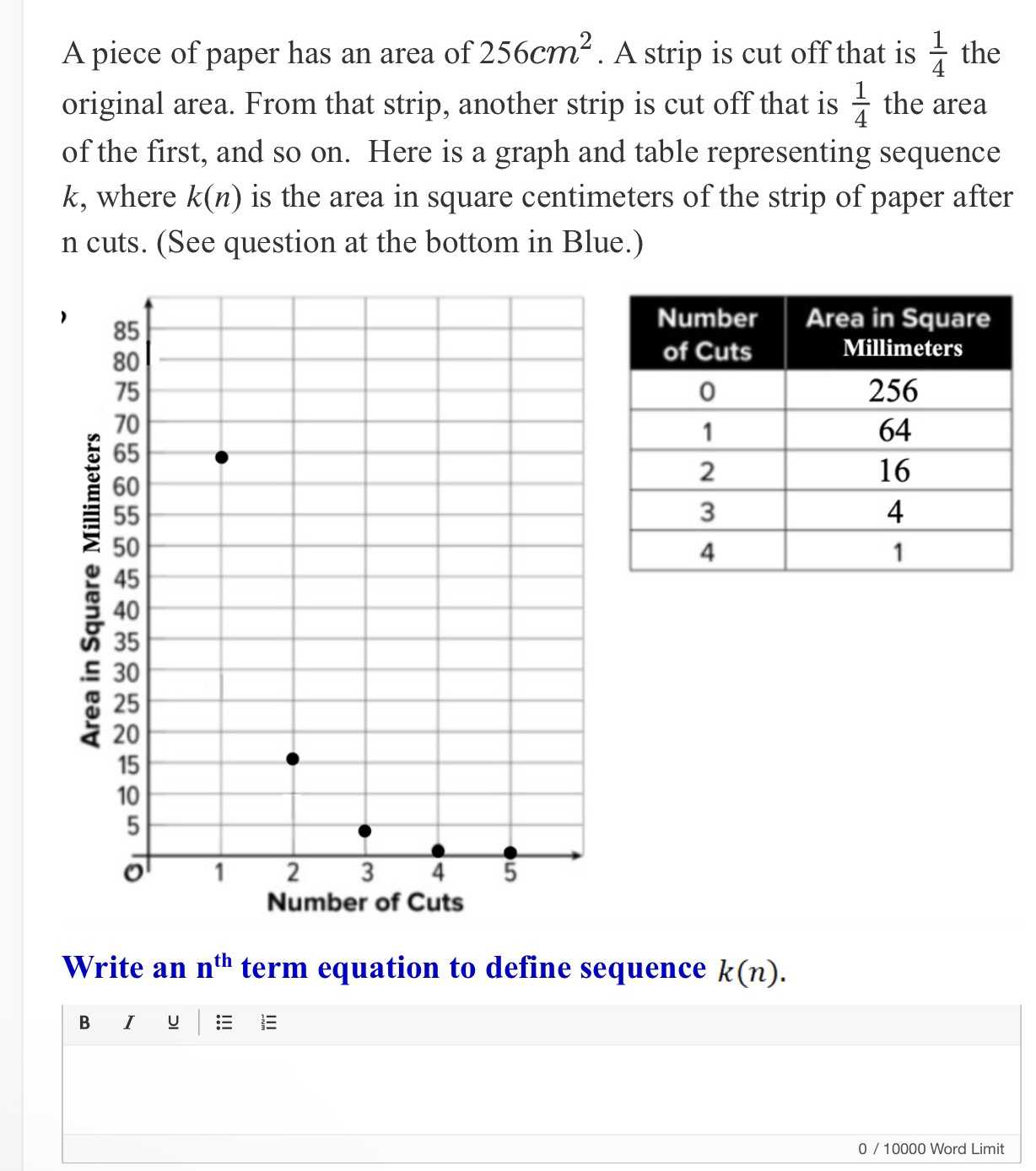### ¿Todavía tienes preguntas de matemáticas?

Pregunte a nuestros tutores expertos
Algebra
PreguntaA piece of paper has an area of $$256 cm ^ { 2 }$$ . A strip is cut off that is $$\frac { 1 } { 4 }$$ the original area. From that strip, another strip is cut off that is $$\frac { 1 } { 4 }$$ the area of the first, and so on. Here is a graph and table representing sequence $$k$$ , where $$k ( n )$$ is the area in square centimeters of the strip of paper after n cuts. (See question at the bottom in Blue.)

Write an $$n^{th}$$term equation to define sequence $$k ( n )$$

$$k(n)= 256\times (\frac{1}{8} )^{n- 1}$$